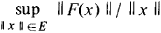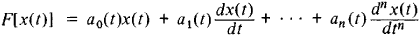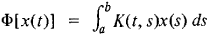# Linear Operator

Also found in: Dictionary, Thesaurus, Wikipedia.
Related to Linear Operator: Linear differential operator

## linear operator

[′lin·ē·ər ′äp·ə‚rād·ər]
(mathematics)
McGraw-Hill Dictionary of Scientific & Technical Terms, 6E, Copyright © 2003 by The McGraw-Hill Companies, Inc.
The following article is from The Great Soviet Encyclopedia (1979). It might be outdated or ideologically biased.

## Linear Operator

a generalization of the concept of linear transformation to vector spaces. F is called a linear operator on a vector space E if it is a function on E with values in some vector space E1 and has the linearity property

F(αx + βy) = αF(x) + βF(y)

where x and y are elements of E, and α and β are numbers. If the E and E1 are normed spaces and ǀǀF(x)ǀǀ / ǀǀxǀǀ is uniformly bounded for all xE, then the linear operator F is said to be bounded andis called its norm.

The most important linear operators on function spaces are the differential linear operatorsand the integral linear operatorsThe Laplace operator is an example of a linear operator on a space of functions of many variables. The theory of linear operators finds numerous applications in various problems of mathematical physics and applied mathematics.

References in periodicals archive ?
We have by Theorem 2.1 that [phi] is an invertible linear operator which preserves commuting pairs of matrices.
Fortunately, the homotopy-series (12) contains an auxiliary parameter h, and besides we have great freedom to choose the auxiliary linear operator L, as illustrated by Liao (20).
 A family of bounded linear operators R(t) [member of] B(X) for t [member of] J is called a resolvent operator for
If T: X [right arrow] Y is a surjective bounded linear operator then T is sequentially continuous.
For a space X, B(X) will denote the set of bounded linear operators on X.
A densely defined linear operator T is called closed if its graph g(T) [subset] H [direct sum] H is closed (, section 46).
is a convolution linear operator on [F.sub.[theta]](N').
The matrix E[a] defines an invertible linear operator.
(iv) When a closed densely defined linear operator A in a complex Banach space X generates a strongly continuous group [{T(t)}.sub.t[member of]R] of bounded linear operators (see, e.g., [3, 5]), i.e., the associated abstract Cauchy problem (ACP)
A bounded linear operator A is said to be quasi-Hermitian if its imaginary component

Site: Follow: Share:
Open / Close